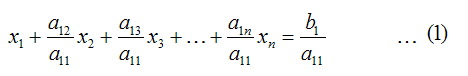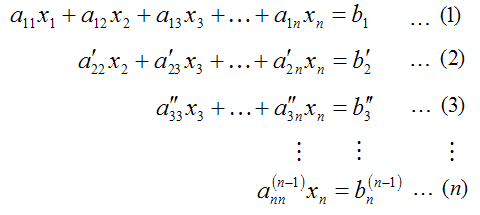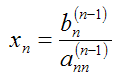Improve math skills of your kids - Learn step-by-step arithmetic from Math games

Math: Unknown - Step-by-step math calculation game for iOS.

Math: Unknown is much more than a math game. It is a step-by-step math calculation game which will teach users how to calculate in the correct order rather than just asking only the final calculated results.

The app consists of four basic arithmetic operations which are addition, subtraction, multiplication and division. In order to get started, users who are new to arithmetic can learn from animated calculation guides showing step-by-step procedures of solving each type of operation. It is also helpful for experienced users as a quick reference.

Generally, addition and subtraction may be difficult for users who just start learning math especially when questions require carrying or borrowing (also called regrouping). The app helps users to visualize the process of carrying and borrowing in the way it will be done on paper. Once users understand how these operations work, they are ready to learn multiplication and division.

For most students, division is considered as the most difficult arithmetic operation to solve. It is a common area of struggle since it requires prior knowledge of both multiplication and subtraction. To help users understand division, the app uses long division to teach all calculation procedures. Relevant multiplication table will be shown beside the question. Users will have to pick a number from the table which go into the dividend. Multiplication of selected number and divisor is automatically calculated, but the users have to do subtraction and drop down the next digit themselves. Learning whole calculation processes will make them master it in no time.

Math: Unknown is a helpful app for students who seriously want to improve arithmetic calculation skills.

Solving System of Equations using Gauss Elimination Method (Part 2)

The the previous post [Solving System of Equations using Gauss Elimination Method (Part 1)], the basic information regarding Gauss Elimination Method has been shared. In this post, we will have at more details about Gauss Elimination method.

Why called "elimination"?

The general form of system of equations is like this.The Gauss Elimination method starts from forward elimination by dividing equation (1) with coefficient of x1. Equation (1) now becomes:Multiply equation (1) with coefficient of x1 from equation (2) then we get:Then we subtract equation (2) with equation (1). Equation (2) becomes:Or we can write it asRepeat the same procedures for the remaining equations and we get the system of equations as follows:We can see that the first term in equation (2) to (n) are eliminated for this round of calculation. For the next round, we will repeat the same procedure, only we change the coefficient to equation (2) i.e. dividing equation (2) with a'22 and multiplying it with a'32 ... As soon as we do the forward elimination until round (n-1) we will get the system of linear equations that is ready for backward substitution as follows.Where: the superscript ', ", ... (n-1) means number of round of forward elimination
We can find from the above system of equations that, first, we can compute the value of xn from equation (n) from:Then we can calculate for xn-1, xn-2, ..., x2, x1 by backward substitution using the following equation.The excel program we developed to solve system of equations using Gauss Elimination method is based on the above equations with some improvements. We will discuss later in the next post about the limitation of Gauss Elimination method with improvement method.